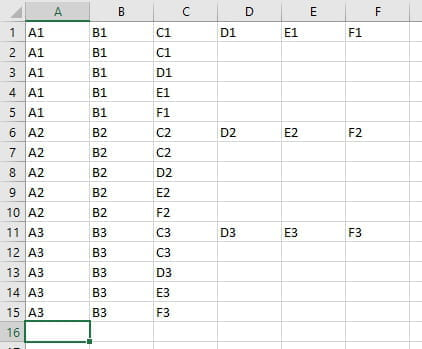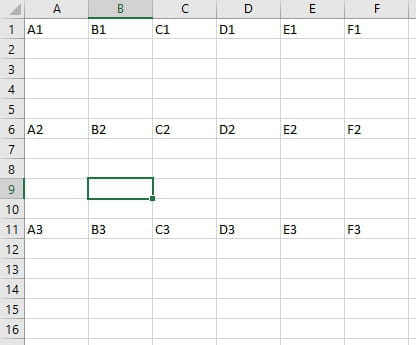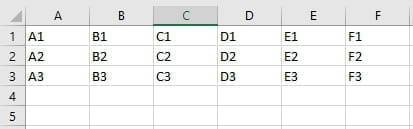# Use of IF

Solved/Closed
Anup27 Posts 2 Registration date Wednesday December 2, 2020 Status Member Last seen February 24, 2021 - Dec 2, 2020 at 08:39 AM
TrowaD Posts 2900 Registration date Sunday September 12, 2010 Status Moderator Last seen September 12, 2022 - Dec 7, 2020 at 12:08 PM
Hello,

Guys I am new to excel but I got a complex situation at work. Hope someone can help.

I want a formula where if I change the date in one column, then the data is divided according to the formula used on those cells.

eg. If date is 1st October 2021, volume in cell A is 60 which is divided in cell B and C as per formula B/12*9 and C/12*3 respectively. But suppose the data changes to 1st December 2021, the data should be divided in cell B and C as B/12*11 and C/12*1 respectively. Dividing basically month vise.

## 2 replies

TrowaD Posts 2900 Registration date Sunday September 12, 2010 Status Moderator Last seen September 12, 2022 523
Dec 3, 2020 at 12:15 PM
Hi Anup,

I find it hard to understand what you are going for.

What I read is that you have a cell with a date in it.
Cell A2 (row 2 as an example) has value 60 and in B2 the formula =B/12*9, which isn't a proper formula. Same goes for C2.

If you want to know how to get the number 9 and 3 when the date is 1st October 2021 located in cell D1 (for example), then try this:
=MONTH(D1)-1 to get to 9
=12-(MONTH(D1)-1) to get to 3

...

Maybe you mean for B2: = A2/12*(MONTH(D2)-1)

Am I close?

Best regards,
Trowa
Hi Trowa,

Yes, it was a mistake and I wanted to divide the volume un A to B and C column. I was able to come up with the same formula. Thank you very much for your quick reply.

But I have now a new challenge. Can you please help me.

Suppose I have one row with data in column A,B,C,D,Eand F.

Now suppose the name in A and B has to be repeated 4 times in 4 rows but C D and E will come in different rows.

Eg I want my one row to be divided in 5 rows with the following combination.

1st row A B C
2nd row A B D
3rd row A B E
4th row A B F

I have like this thousands of rows for which I would like to then repeat this formula so that same thing happens to every different row

Best Regards,
Anup
TrowaD Posts 2900 Registration date Sunday September 12, 2010 Status Moderator Last seen September 12, 2022 523
Dec 7, 2020 at 12:08 PM
Hi Anup,

So you want you end result to look like this:It not clear to me though, what you starting situation looks like.

If it looks like this:Then use this code:
```Sub RunMe()
Dim x, lRow As Long
lRow = Range("A" & Rows.Count).End(xlUp).Row
For x = 1 To lRow Step 5
Range("A" & x, "B" & x).Copy Range("A" & x + 1, "B" & x + 4)
Range("C" & x, "F" & x).Copy
Range("C" & x + 1).PasteSpecial Transpose:=True
Next x
Application.CutCopyMode = False
End Sub```

When your data looks like this:Then use this code:
```Sub RunMe()
Dim x, lRow As Long
lRow = Range("A" & Rows.Count).End(xlUp).Row
For x = 1 To lRow * 5 Step 5
Rows(x + 1 & ":" & x + 4).Insert
Range("A" & x, "B" & x).Copy Range("A" & x + 1, "B" & x + 4)
Range("C" & x, "F" & x).Copy
Range("C" & x + 1).PasteSpecial Transpose:=True
Next x
Application.CutCopyMode = False
End Sub```

If you are unfamiliar with codes then read the following:
How to implement and run a code:

- From Excel hit Alt + F11 to open the “Microsoft Visual Basic” window.
- Go to the top menu in the newly opened window > Insert > Module.
- Paste the code in the big white field.
- You can now close this window.
- Back at Excel, hit Alt + F8 to display the available macro’s.
- Double-click the macro you wish to run.
NOTE: macro’s cannot be reversed using the blue arrows. Always make sure you save your file (or create a back up to be entirely sure) before running a code, so you can re-open your file if something unforeseen happens or you want to go back to the situation before the code was run.

Best regards,
Trowa